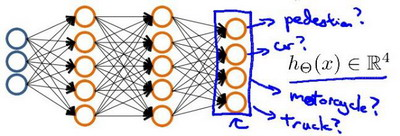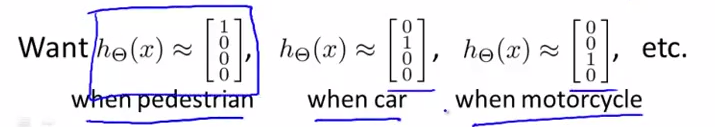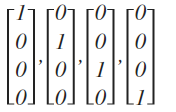# 第4周

## 第八、神经网络：表述(Neural Networks: Representation)

### 8.1 非线性假设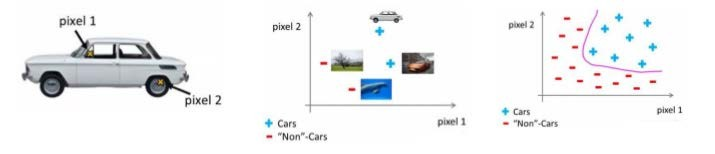### 8.2 神经元和大脑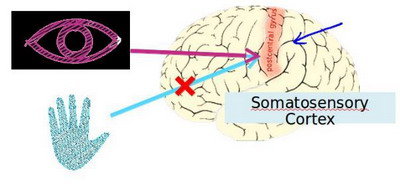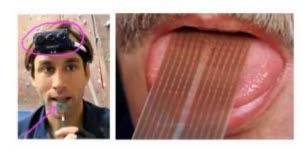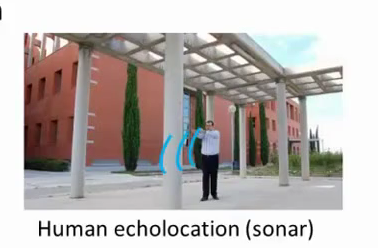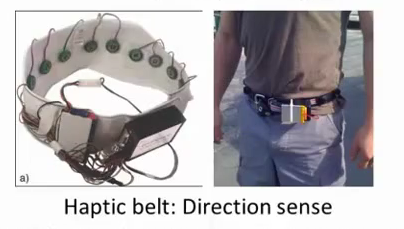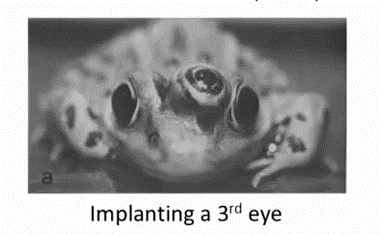### 8.3 模型表示1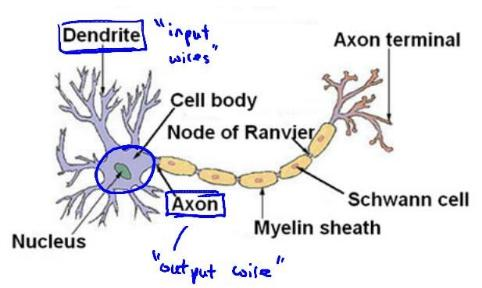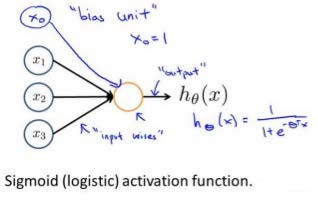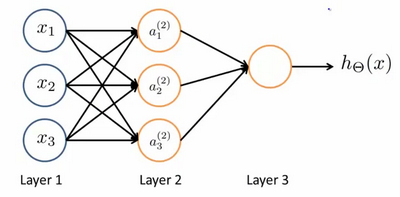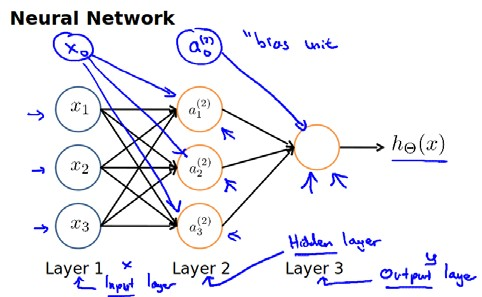$a_{1}^{(2)}=g(\Theta _{10}^{(1)}{{x}_{0}}+\Theta _{11}^{(1)}{{x}_{1}}+\Theta _{12}^{(1)}{{x}_{2}}+\Theta _{13}^{(1)}{{x}_{3}})$ $a_{2}^{(2)}=g(\Theta _{20}^{(1)}{{x}_{0}}+\Theta _{21}^{(1)}{{x}_{1}}+\Theta _{22}^{(1)}{{x}_{2}}+\Theta _{23}^{(1)}{{x}_{3}})$ $a_{3}^{(2)}=g(\Theta _{30}^{(1)}{{x}_{0}}+\Theta _{31}^{(1)}{{x}_{1}}+\Theta _{32}^{(1)}{{x}_{2}}+\Theta _{33}^{(1)}{{x}_{3}})$ ${{h}_{\Theta }}(x)=g(\Theta _{10}^{(2)}a_{0}^{(2)}+\Theta _{11}^{(2)}a_{1}^{(2)}+\Theta _{12}^{(2)}a_{2}^{(2)}+\Theta _{13}^{(2)}a_{3}^{(2)})$

（我们把这样从左到右的算法称为前向传播算法( FORWARD PROPAGATION )）

$x$, $\theta$, $a$ 分别用矩阵表示：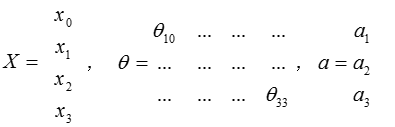### 8.4 模型表示2

( FORWARD PROPAGATION ) 相对于使用循环来编码，利用向量化的方法会使得计算更为简便。以上面的神经网络为例，试着计算第二层的值：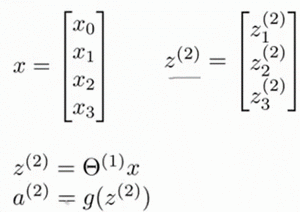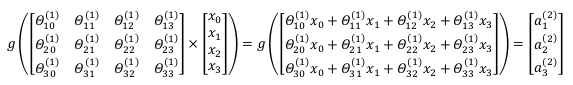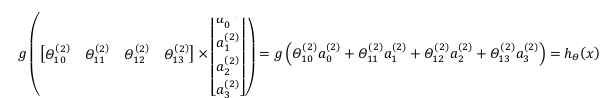${{a}^{\left( 2 \right)}}=g({{z}^{\left( 2 \right)}})$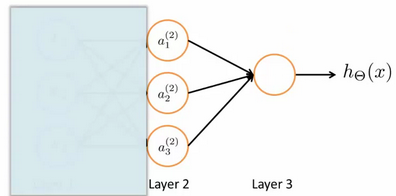### 8.5 特征和直观理解1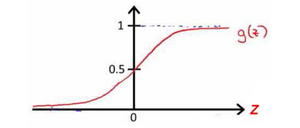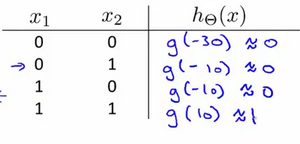ORAND整体一样，区别只在于的取值不同。

### 8.6 样本和直观理解II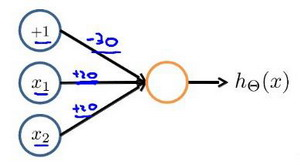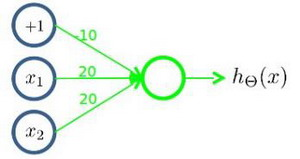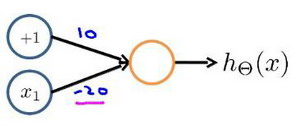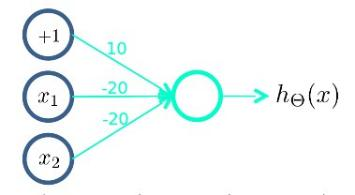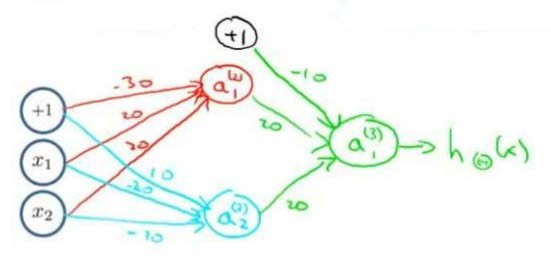### 8.7 多类分类